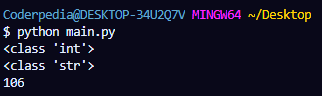Python String to Int and Int to String: In Python, you can directly convert one data type into another. In this article, you will learn to convert String to Integer and Integer to String.

During your programming or software development journey, you will need to convert one data type into another.

For example – While reading numeric data from another file, the data will be in String format. So, you have to explicitly convert it into Integer type to perform mathematical operations on that data.

So, today we will take a deep look at different methods which you can use to convert String to Integer data type and Integer to String data type.

However, first let’s see the different ways to store or represent Integer in Python.

## Representing Integer using str and int

In Python, you can store Integer value using different data types. Some of the most common data types used to store Integer are str and int.

For example – You can represent integer value 150 using String literal in Python as follow:

s_value = “150”

Similarly, To represent integer value 150 using Integer literal in Python. Use the following code.

i_value = 150

Now, these are the Decimal representation of the Integer value.

Similarly, there are other Number System available, like – Binary Number System, Octal Number System and Hexadecimal Number System.

In Python, you can easily represent the Integer in these Number System by using prefix.

You have to add some specific prefix to represent these Number System in Integer.

For example – To represent Binary value(10010110) in Integer(106), add “0b” prefix.

b_value = 0b1101010

To represent Octal value(226) in Integer(106), add “0o” prefix.

o_value = 0o152

h_value = 0x6A

You can also represent these Values in String literal by using quote(” “) symbol.

```				```
b_value = "0b1101010"
o_value = "0o152"
h_value = "0x6A"
```
```

However, to print the associated Integer values of it, you have to explicitly convert these String values to Integer, which we have discussed below.

## Problem without Type Conversion

Before jumping into the solution, let’s take a look at the problems which you will face without using type conversation in Python.

In Programming, many times we have to use String and Integer value in the same statement.

However, you can’t concatenate String value and Integer value directly in Python.

Note: In Python, type() is used to get the Data Type of the variable.

For example –

```				```
marks = 85
print(type(marks))

print("Sam got " + marks + " marks in Mathematics")
```
```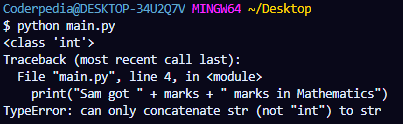As a result, you will get a TypeError. You have to explicitly convert marks to String type.

Similarly, if you add two variables of int and string type.

```				```
i_value = 95
print(type(i_value))

s_value = "100"
print(type(s_value))

print(s_value + i_value)
```
```

You will again get a TypeError. As you have to explicitly convert s_value to Int type.

## How to Convert String to Int in Python

There are 3 different ways which you can use to convert a String to Int in Python:

### 1. Converting String to Int using int()

To convert the String to Int and solve the above discussed problem in Python, you can make use of int() method.

It is used to convert value of String type to Integer type.

```				```
i_value = 95
print(type(i_value))

s_value = "100"
print(type(s_value))

print(int(s_value) + i_value)
```
```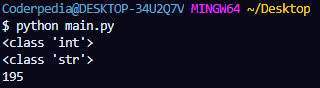After the conversion of s_value to Int type, we get result as 195.

### 2. Converting String to Int from different base

Now as we have discussed above, there are different Number System which are used in Python, each having different base value.

For example – Binary Number have base 2, Octal Number have base 8 and Hexadecimal Number have base 16.

Now, if you store these numbers as String literal and you want to print the Integer value of these Numbers, then you can’t do it directly.

```				```
b_value = "0b1101010"
print(type(b_value))

o_value = "0o152"
print(type(o_value))

h_value = "0x6A"
print(type(h_value))

print(b_value)
print(o_value)
print(h_value)
```
```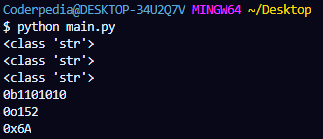As you can see, we can’t print the Integer value of these variables directly, as they are of String type.

So, to convert these values in Integer type, you have to use the int() and the respective base value associated with it.

```				```
b_value = "0b1101010"
b_value = int(b_value, base = 2)
print(type(b_value))

o_value = "0o152"
o_value = int(o_value, base = 8)
print(type(o_value))

h_value = "0x6A"
h_value = int(h_value, base = 16)
print(type(h_value))

print(b_value)
print(o_value)
print(h_value)
```
```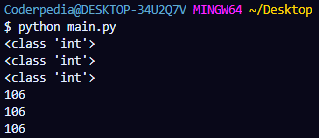Best Course

-> Best Course for learning Python: Python for Everybody Specialization

### 3. Converting Character to Int using ord()​​​

In programming, many times we want to access the unicode value of the characters.

For example – The unicode value of ‘A’ is 65 and unicode value of ‘m’ is 109.

So, In Python to access the unicode value of any character, you can use ord() method.

The Python code example to convert Character to Int using ord() is

```				```
n = ord('A')
m = ord('c')

print(n)
print(m)
```
```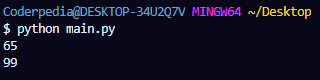## How to convert Int to String in Python

As we have converted String to Int, similarly we can convert Int to String using different ways in Python:

### 1. Converting Int to String

It becomes very easy to convert Int to String in Python with the help of str().

For example – The String value and Integer value can be concatenated as follow:

```				```
marks = 85
print(type(marks))

marks = str(marks)
print(type(marks))

print("Sam got " + marks + " marks in Mathematics")
```
```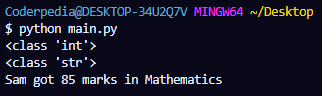### 2. Converting Hexadecimal to String​​​​

In Python, by default Hexadecimal String is automatically represented in a Decimal Number System.

For example –

```				```
h_value = "0x6A"
print(type(h_value))

print(h_value)
```
```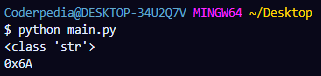You can explicitly convert Hexadecimal to String using str() as well.

```				```
h_value = "0x6A"
print(type(h_value))

h_value = str(h_value)
print(type(h_value))

print(h_value)
```
```### 3. Converting Octal to String

Similarly, you can also convert Octal to String using str() in Python.

```				```
o_value = 0o152
print(type(o_value))

o_value = str(o_value)
print(type(o_value))

print(o_value)
```
```